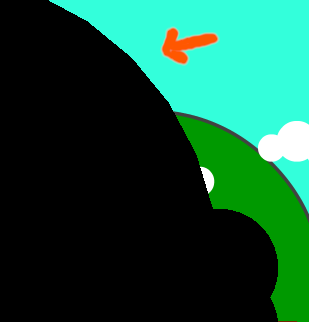+1 vote

Hi,

I'm trying to create a transition between 2 scenes using an image created by a combination of draw_X() functions.
All is working well, except when I need to draw a large circle (radius 1080/2). It doesn't look very smooth at all ...Is there a way to fix this ?
Thanks !

in Engine

There was a suggestion by me and Godot contributor to have this implemented internally, but here's a version in GDScript that does it for now:

``````extends Node2D

return

var maxpoints = 1024 # I think this is renderer limit

var numpoints = ceil(PI / acos(1.0 - maxerror / radius))
numpoints = clamp(numpoints, 3, maxpoints)

var points = PoolVector2Array([])

for i in numpoints:
var phi = i * PI * 2.0 / numpoints
var v = Vector2(sin(phi), cos(phi))

draw_colored_polygon(points, Color(1.0, 1.0, 1.0))

func _draw():
draw_circle_custom(1000)
``````

The result should be pretty smooth already. You can experiment with `maxerror` parameter to fine tune the smoothness/performance. This can be even be hacked to draw hexagons by specifying high `maxerror` value!

by (1,378 points)
edited by

Ah ! Perfect ! Thank you !

I hope this will be implemented in some future version of Godot. And preferrably also with a way to specify its position. Otherwise, how could you use this in combination with other draw calls such as draw_circle(position, radius, color) ???

You can actually offset drawing with:
`void draw_set_transform( Vector2 position, float rotation, Vector2 scale )`

From the docs:

Sets a custom transform for drawing via components. Anything drawn afterwards will be transformed by this.

``````func _draw():
draw_circle_custom(100) # at Vector2(0, 0) relative to canvas item
draw_set_transform(Vector2(200, 0), 0.0, Vector2(1.0, 1.0))`
draw_circle_custom(100) # will be drawn with offset 200 pixels right ->
``````

Or you could just draw the circle on a separate canvas item (`Node2D`) as child of the root scene, and simply set it's position/transform with corresponding property.

Think of drawing as of commands that are passed to the rendering engine, these commands are then interpreted and drawn all in order.

Interesting !

Seems like you really can do just about anything with the Godot Engine. =)

As far as I know there's now draw_circle() function. So you probably created some own function that draws a circle.

If you e.g. did a function `draw_circle_arc` similar to that from the docs:
https://docs.godotengine.org/en/latest/tutorials/2d/custom_drawing_in_2d.html?highlight=draw

Then you might want to increase the nb_points value to have a better approximation to a circle.

by (3,358 points)

Yes, I used the draw_circle() function, which is fine for smaller circles. Large circles however have jagged edges (look like a polygon instead of a circle).

I'll look into the example code for 'Arc polygon function' like you suggested. Hopefully that will work.

Thanks for helping !

Oh, I see. I missed that there actually exists a `draw_circle` function. My fault.

No worries. We both learned something new. =)